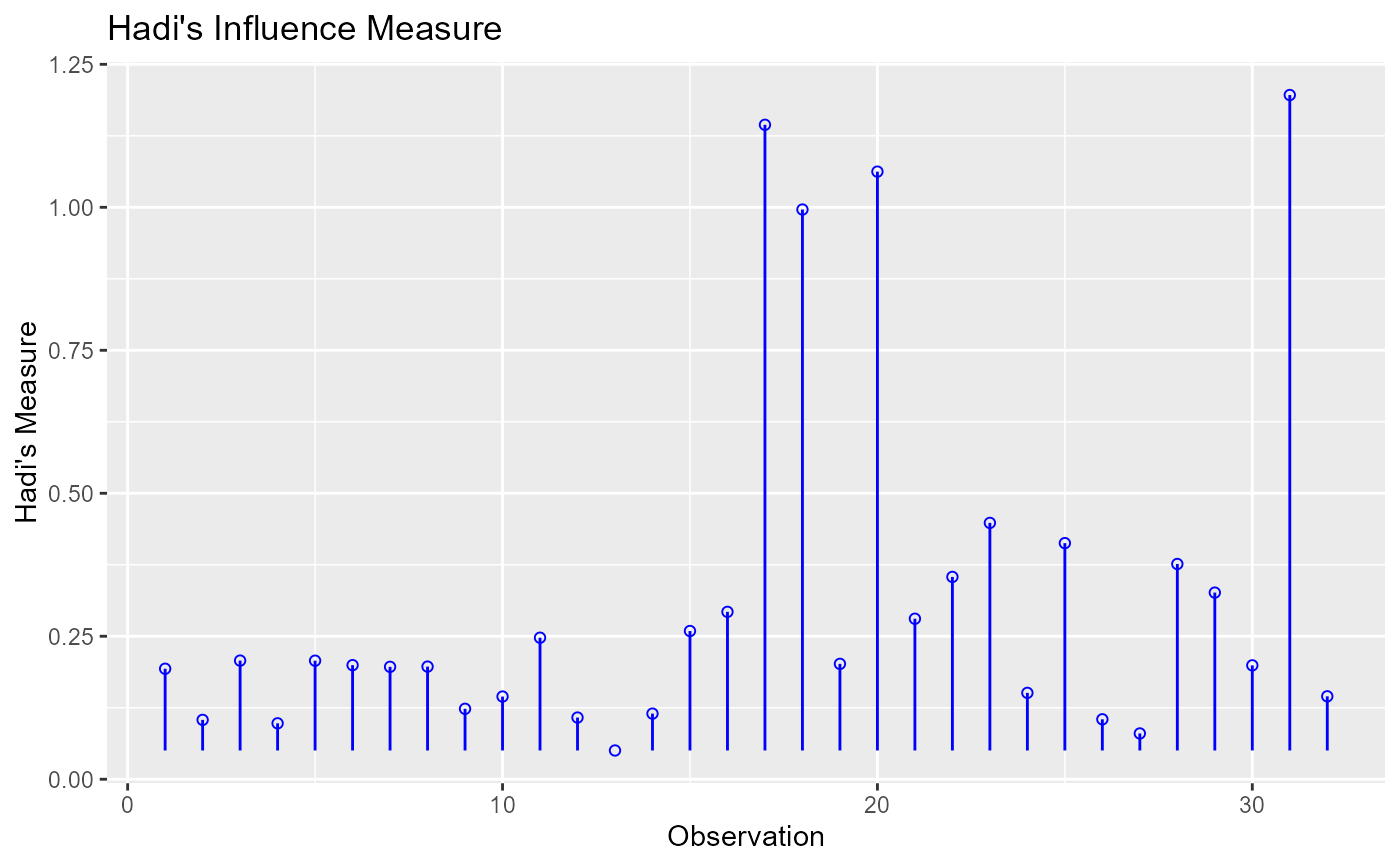Hadi's measure of influence based on the fact that influential observations can be present in either the response variable or in the predictors or both. The plot is used to detect influential observations based on Hadi's measure.

ols_plot_hadi(model, print_plot = TRUE)

## Arguments

model An object of class lm. logical; if TRUE, prints the plot else returns a plot object.

## Deprecated Function

ols_hadi_plot() has been deprecated. Instead use ols_plot_hadi().

Chatterjee, Samprit and Hadi, Ali. Regression Analysis by Example. 5th ed. N.p.: John Wiley & Sons, 2012. Print.

model <- lm(mpg ~ disp + hp + wt, data = mtcars)
ols_plot_hadi(model)